# Gas constant

Values of R Units
(V·P·T-1·n-1)
8.314472 J·K-1·mol-1
0.0820574587 L·atm·K-1·mol-1
8.20574587 × 10-5 m3·atm·K-1·mol-1
8.314472 cm3·MPa·K-1·mol-1
8.314472 L·kPa·K-1·mol-1
8.314472 m3·Pa·K-1·mol-1
62.36367 L·mmHg·K-1·mol-1
62.36367 L·Torr·K-1·mol-1
83.14472 L·mbar·K-1·mol-1
1.987 cal·K-1·mol-1
6.132440 lbf·ft·K-1·g-mol-1
10.73159 ft3·psi· °R-1·lb-mol-1
0.7302413 ft3·atm·°R-1·lb-mol-1
998.9701 ft3·mmHg·K-1·lb-mol-1
8.314472 × 107 erg·K-1·mol-1

The gas constant (also known as the molar, universal, or ideal gas constant) is a physical constant that is featured in a number of fundamental equations in the physical sciences, such as the ideal gas law and the Nernst equation. It is expressed in units of energy (that is, the pressure-volume product) per kelvin per mole. It is equivalent to the Boltzmann constant, except that the latter is expressed in units of energy per kelvin per particle.

Denoted by the symbol R, the value of the gas constant is:

R = 8.314472(15) J · K-1 · mol-1

The two digits in parentheses indicate the uncertainty (standard deviation) in the last two digits of the value.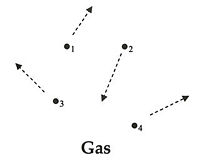Movement of particles (atoms, molecules, or ions) in the gas phase. The gas constant is a factor in the ideal gas law, which provides an approximation of some of the properties of gases.

## Ideal gas law

An ideal gas (or "perfect" gas) is a hypothetical gas consisting of a very large number of identical particles, each of zero volume, uniformly distributed in density, with no intermolecular forces. Additionally, the molecules or atoms of the gas have complete randomness of direction and velocity, and they undergo perfectly elastic collisions with the walls of the container. The molecules of an ideal gas are often compared to rigid but elastic billiard balls.

The gas constant occurs in the ideal gas law (the simplest equation of state) as follows: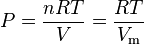$P = \frac{nRT}{V} = \frac{RT}{V_{\rm m}}$

where:$P\,\!$ is the absolute pressure$T\,\!$ is absolute temperature$V\,\!$ is the volume the gas occupies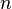$n\,\!$ is the amount of gas (in terms of the number of moles of gas)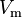$V_{\rm m}\,\!$ is the molar volume

This equation does not exactly apply to real gases, because each molecule of a real gas does occupy a certain volume and the molecules are subject to intermolecular forces. Nonetheless, this equation is used as an approximation when describing the behavior of a real gas, except when the gas is at high pressures or low temperatures.

## Relationship with the Boltzmann constant

The Boltzmann constant kB (often abbreviated k) has the value 1.3807 x 10-23 J.K-1. It may be used in place of the universal gas constant by working in pure particle count, N, rather than number of moles, n, since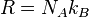$\qquad R=N_A k_B\,\!$,

where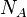$N_A$ is Avogadro's number (= 6.022 x 1023 particles per mole).

In terms of Boltzmann's constant, the ideal gas law may be written as: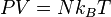$PV=Nk_BT\,\!$

where N is the number of particles (atoms or molecules) of the ideal gas.

Given its relationship with the Boltzmann constant, the ideal gas constant also appears in equations unrelated to gases.

## Specific (or individual) gas constant

The specific gas constant or individual gas constant of a gas or mixture of gases (Rgas or just R) is given by the universal gas constant, divided by the molar mass ($M$) of the gas or mixture. Whereas the universal gas constant is the same for all ideal gases, the specific (or individual) gas constant is applicable to a particular gas (or mixture of gases such as air).

The equation to calculate the specific gas constant for a particular gas is as follows:$R_{\rm gas} = \frac{\bar{R}}{M}$

where: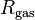$R_{\rm gas}\,\!$ is the specific gas constant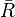$\bar{R}$ is the universal gas constant$M\,\!$ is the molar mass (or molecular weight) of the gas

In the SI system, the units for the specific gas constant are J·kg-1·K-1; and in the imperial system, the units are ft·lb·°R-1·slug-1.

The specific gas constant is often represented by the symbol R, and it could then be confused with the universal gas constant. In such cases, the context and/or units of R should make it clear as to which gas constant is being referred to. For example, the equation for the speed of sound is usually written in terms of the specific gas constant.

The values of the individual gas constant for air and some other common gases are given in the table below.

Gas Individual Gas Constant
SI Units
(J·kg-1·K-1)
Individual Gas Constant
Imperial Units
(ft·lb·°R-1·slug-1)
Air 286.9 1,716
Carbon dioxide (CO2) 188.9 1,130
Helium (He) 2,077 12,420
Hydrogen (H2) 4,124 24,660
Methane (CH4) 518.3 3,099
Nitrogen (N2) 296.8 1,775
Oxygen (O2) 259.8 1,554
Water vapor (H2O) 461.5 2,760

## US Standard Atmosphere

The US Standard Atmosphere, 1976 (USSA1976) defines the Universal Gas Constant as:$\bar{R} = 8.31432\times 10^3 \frac{\mathrm{N \cdot m}}{\mathrm{kmol \cdot K}}$

The USSA1976 does recognize, however, that this value is not consistent with the cited values for the Avogadro constant and the Boltzmann constant. This disparity is not a significant departure from accuracy, and USSA1976 uses this value of R for all the calculations of the standard atmosphere. When using the ISO value of R, the calculated pressure increases by only 0.62 pascals at 11,000 meters (the equivalent of a difference of only 0.174 meters, or 6.8 inches) and an increase of 0.292 pascals at 20,000 meters (the equivalent of a difference of only 0.338 meters, or 13.2 inches).